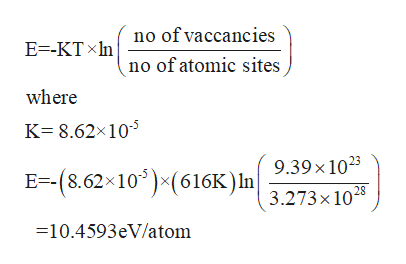# Calculate the energy (in eV/atom) for vacancy formation in some metal, M, given that the equilibrium number of vacancies at 343oC is 9.39 × 1023 m-3. The density and atomic weight (at 343°C) for this metal are 7.75 g/cm3 and 142.6 g/mol, respectively.

Question
44 views

Calculate the energy (in eV/atom) for vacancy formation in some metal, M, given that the equilibrium number of vacancies at 343oC is 9.39 × 1023 m-3. The density and atomic weight (at 343°C) for this metal are 7.75 g/cm3 and 142.6 g/mol, respectively.

check_circle

Step 1

Given

Temperature of metal= 343°C= (343+273)K=616K

Density of the metal= 7.75g/cc=7.75x106g/m3

Atomic mass = 142.6g/mol

Vacancies = 9.39x 1023/m3

Step 2

The number of atomic sites

Step 3

The formula for the energy of formation of v...help_outlineImage Transcriptioncloseno of vaccancies no of atomic sites E -KT xIn where K 8.62x10 9.39 x 1023 E-(8.62x10(616K ) In| 3.273x 1023 =10.4593eV/atom fullscreen

### Want to see the full answer?

See Solution

#### Want to see this answer and more?

Solutions are written by subject experts who are available 24/7. Questions are typically answered within 1 hour.*

See Solution
*Response times may vary by subject and question.
Tagged in

### Classification of solids